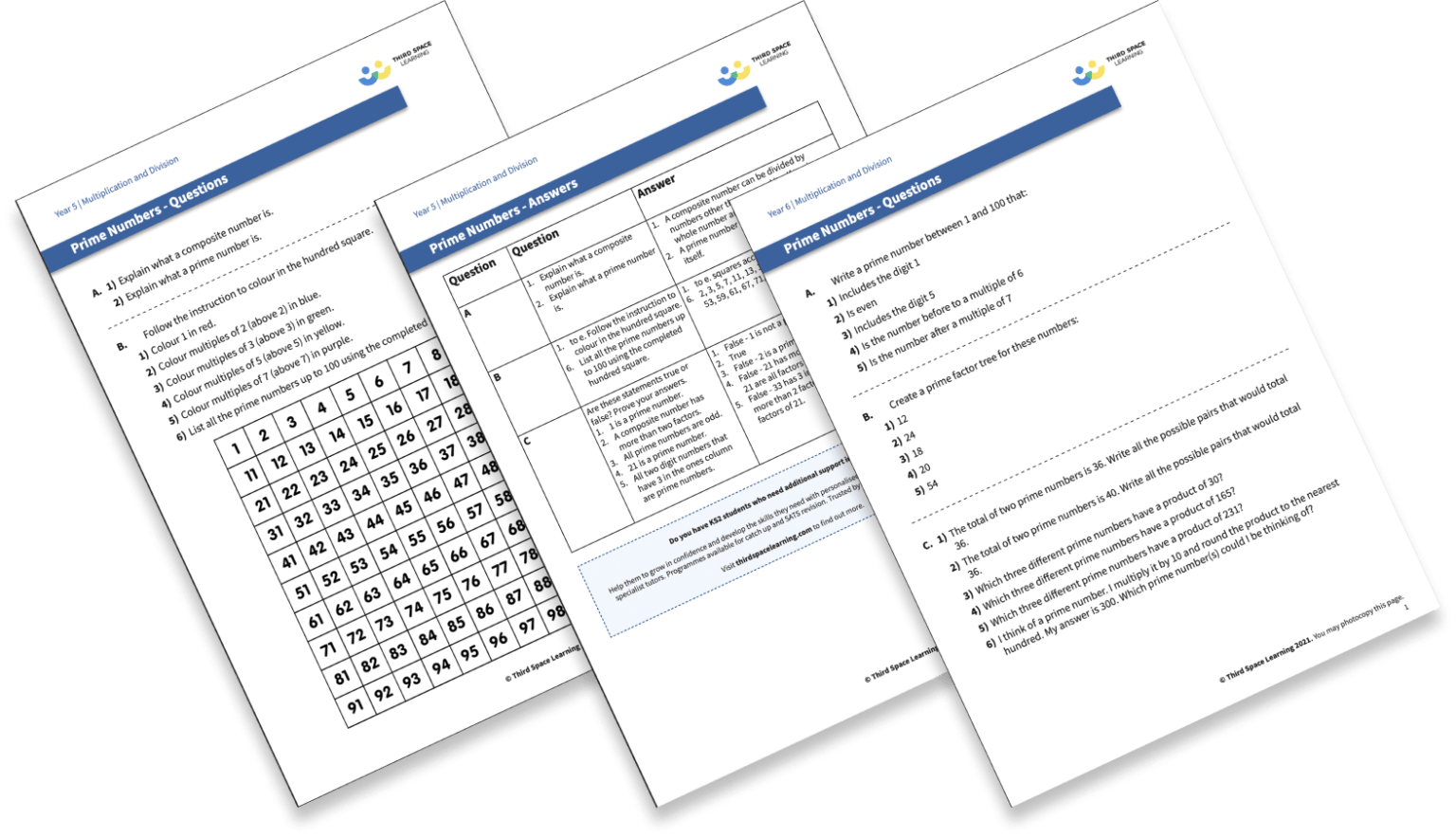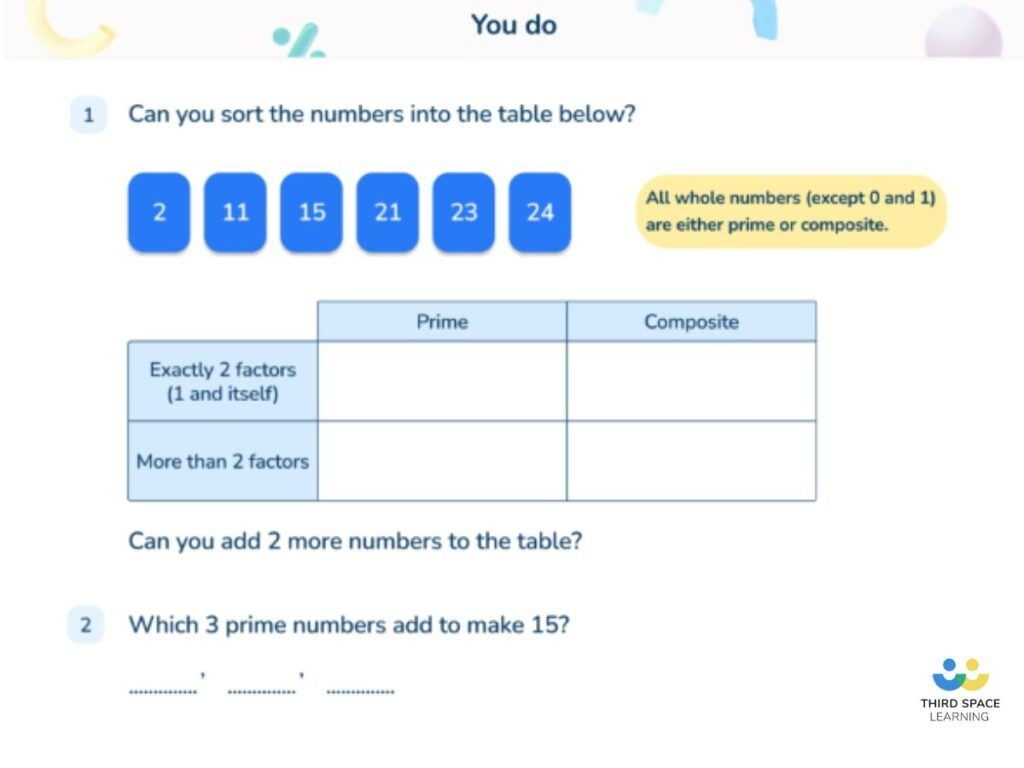Teaching support from the UK’s largest provider of in-school maths tuitionone to one lessonsschools supported

Build mathematical fluency

Give your pupils the opportunity to regularly practise their skills in a nurturing one to one environment

See it in action

Hundreds of FREE online maths resources!

Daily activities, ready-to-go lesson slides, SATs revision packs, video CPD and more!Explore all resources
Fractions, Decimals & Percentages

Build mathematical fluency

Give your pupils the opportunity to regularly practise their skills in a nurturing one to one environment

See it in action

# What Are Composite Numbers? Explained For Primary School Parents & Teachers

Composite numbers are the inverse of prime-numbers. Here we explain what exactly this section of number theory means, tell you how it is used and learnt in primary schools and provide some examples of questions involving composite numbers.

Pupils officially encounter prime numbers and composite numbers in Year 5, and will be expected to work within the range of 1 to 100.

### What is a composite number?

A prime number can only have 2 factors, 1 and the number itself. 3 would be a prime number because its only factors are 1 and 3.

A composite number, on the other hand, can be any whole number that has more than 2 factors. It can be an even or odd number.

Remember that a composite number can be any given number which is a positive integer (or natural number) whose divisors are smaller positive integers, provided the number has a minimum of 3 factors.

Note that a highly composite number has a large number of factors, and outlining this concept to pupils may help them remember that a composite number has multiple factors in comparison to a prime number which just has two factors.

Examples of composite numbers include:

• 4, as it has three factors: 1,2 and 4.
• There are 5 composite numbers between 10: 4, 6, 8, 9, 10

1 is neither a prime nor composite number.FREE Prime and Composite Numbers Worksheets

A FREE resource including 29 questions for your Year 5 and 6 pupils, to help test their ability for working with prime numbers.Download Free Now!

### Composite numbers in primary school

The term ‘composite numbers’ is not introduced formally in the UK until Year 5.

According to the national curriculum, Year 5 children should be taught to:

“know and use the vocabulary of prime numbers, prime factors and composite (non-prime) numbers to establish whether a number up to 100 is prime and recall prime numbers up to 19.”

Students will not be asked to identify numbers as composite outside of the context of discussing prime numbers.

### Composite numbers between 1 and 100

The composite numbers between 1 and 100 are…

4, 6, 8, 9, 10, 12, 14, 15, 16, 18, 20, 21, 22, 24, 25, 26, 27, 28, 30, 32, 33, 34, 35, 36, 38, 39, 40, 42, 44, 45, 46, 48, 49, 50, 51, 52, 54, 55, 56, 57, 58, 60, 62, 63, 64, 65, 66, 68, 69, 70, 72, 74, 75, 76, 77, 78, 80, 81, 82, 84, 85, 86, 87, 88, 90, 91, 92, 93, 94, 95, 96, 98, 99, 100.

Students would not be expected to learn this list off by heart. Using divisibility tests to find factors of a number is very useful in determining whether a number is prime or composite.

Pupils need to know how to find prime numbers up to 100 and know prime numbers up to 19. Primality tests, such as the sieve of eratosthenes or simply trial division can be methods pupils use to figure out which numbers are prime up to a number n, as pupils will be working within a range of small numbers.

Therefore, it is easier to find the prime numbers and know that numbers that are not prime are called composite numbers.Introducing the concept of composite numbers to Year 5 pupils on Third Space Learning’s online intervention.

### How does this relate to other areas of mathematics?

Outside of identifying numbers as prime or composite, it is unlikely that students will be required to know that numbers are composite. However, the application of composite numbers is used throughout mathematics to describe shape, measure, to quantify amounts of objects and to perform arithmetic.

### How does this relate to real life?

Unless working in cyber security (where prime numbers are important) or as a teacher, you are unlikely to come across the term ‘composite numbers’. However,  the application of composite numbers, i.e. any whole number that is not prime is used throughout mathematics (see above).

### Composite numbers worked example

Q. Place these numbers into the table below.

2,3,4,5,6,7,8,9,10

Students should have memorised the prime numbers up to and including 19. They could use this to write the prime numbers into the correct section and then deduce that the other numbers are composite.

For students who do not know the prime numbers up to 19, they could find the factors of each number by using their multiplication facts.

2 = 2 x 1 (2 factors)

3 = 3 x 1 (2 factors)

4 = 4 x 1 = 2 x 2 (3 factors)

### Composite numbers example questions

1. What is the definition of a composite number?

(Answer: A number with more than 2 factors)

2. Which of the following are composite numbers? 51, 97, 69, 57, 53, 90

3. Andy makes the number 17 out of a prime number and a composite number only using addition. What could the numbers be?

(Answer: 2 + 15, 3 + 14, 5 + 12, 7 + 10, 11 + 6, 13 + 4)

4. What is the product of the first 3 composite numbers?

5. Chen chooses a composite number. He multiplies it by 10 and then rounds it to the nearest hundred. His answer is 300. Write all the possible composite numbers Chen could have chosen.

(Answer: 25, 26, 27, 28, 30, 32, 33, 34)

Wondering about how to explain other key maths vocabulary to your children? Check out our Primary Maths Dictionary, or try these:

You can find plenty of worksheets for primary school pupils on the Third Space Learning Maths Hub.

Online 1-to-1 maths lessons trusted by schools and teachers
Every week Third Space Learning’s maths specialist tutors support thousands of primary school children with weekly online 1-to-1 lessons and maths interventions. Since 2013 we’ve helped over 150,000 children become more confident, able mathematicians. Learn more or request a personalised quote to speak to us about your needs and how we can help.

Primary school tuition targeted to the needs of each child and closely following the National Curriculum.

Related Articles

x#### FREE Prime and Composite Numbers Worksheets

A FREE resource including 29 questions for your Year 5 and 6 pupils, to help test their ability for working with prime numbers.

#### FREE Prime and Composite Numbers WorksheetsA FREE resource including 29 questions for your Year 5 and 6 pupils, to help test their ability for working with prime numbers.